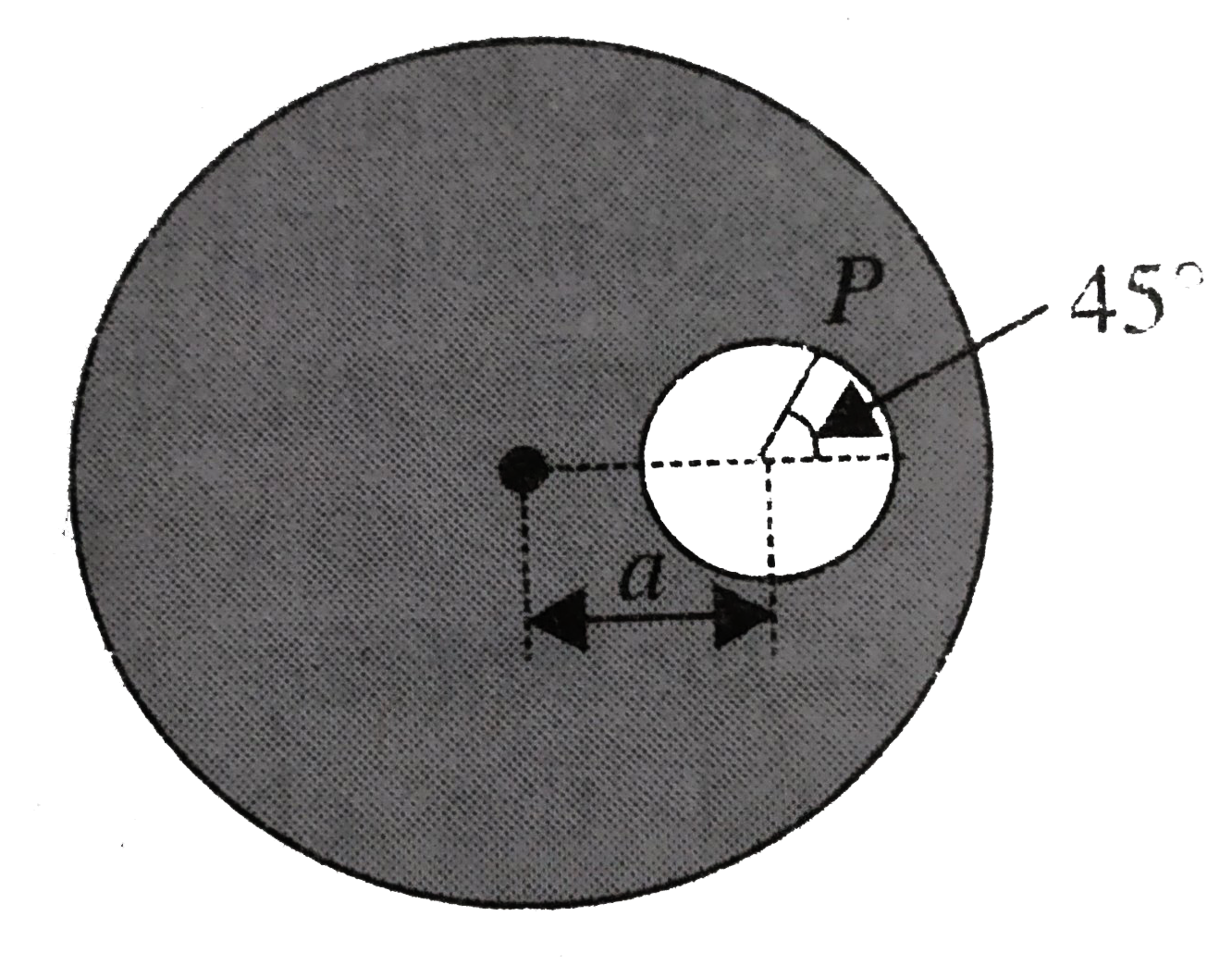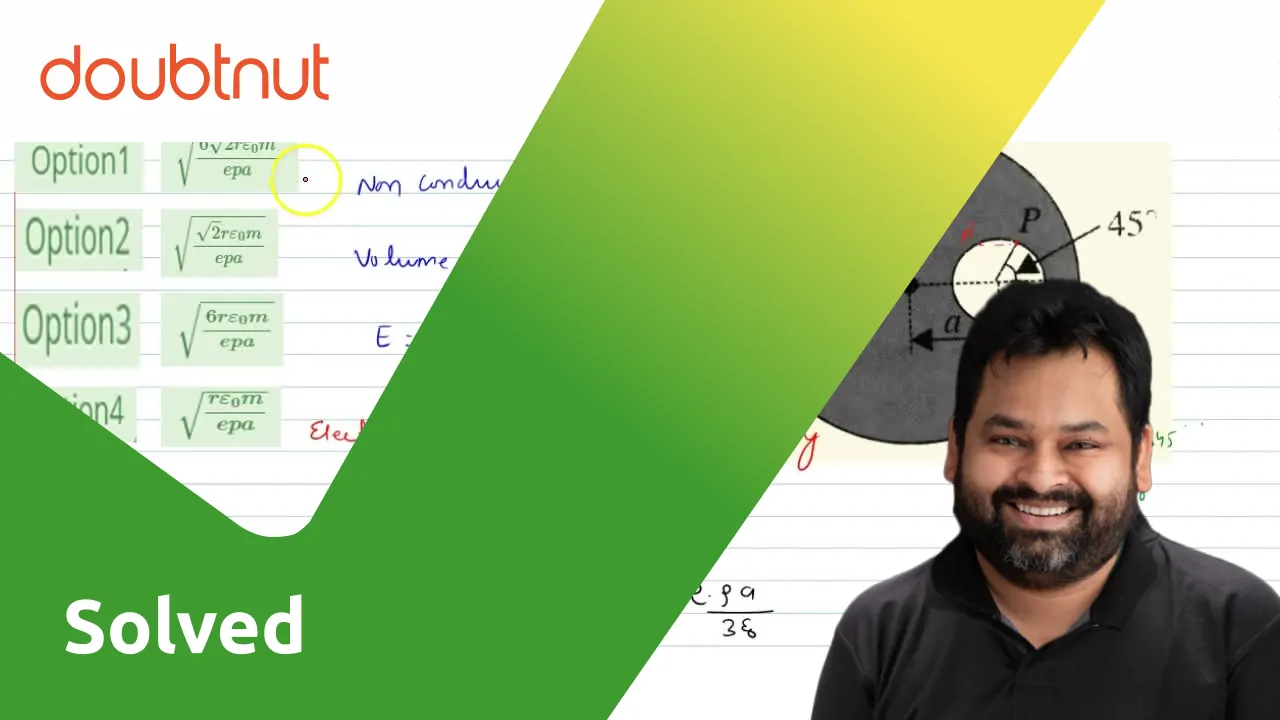Doubtnut App - Get 50 Lakh+ video solutions better than GoogleInstall Now

# A cavity of radius r is made inside a solid sphere. The volume charge density of the remaining sphere is ρ. An electron (charge e, mass m) is released inside the cavity from point P as shown in figure. The centre of sphere and center of cavity are separated by a distance a. The time after which the electron again touches the sphere isA

62rε0mepa

B

2rε0mepa

C

6rε0mepa

D

rε0mepa

Text Solution
Verified by Experts

## Torque due to →B = Torque of weight of (QRST) (about QT) L(B0IL)=3(λL)g(L2+L6)=3λLg(2L3) B0=2λgI No external force, so COM cannot displace initial coordinate of COM=3(λL)(zero)+2λL(L2)+λL(L)6λg=L3 Final coordinate of COM=L3(same) But COM displaces with respect to QT by 2L3. So displacement of QPUT=2L3. Initial magnetic dipole moment M makes an angle of π4 ACW from +ive x-axis and finally it makes angle 3π4 ACW from +ive x-axis(M=√2IL2) So change in PE=−MB(cosθ2−cosθ1)=MB0[cos135∘−cos45∘]=2B0IL2=Gain in KE=12Iω2 I(about QT)=2[2λL33+λL3]=10λL33,2B0IL2=12(Iω2)=12(10λL33)ω2,ω2=6B0I5λL